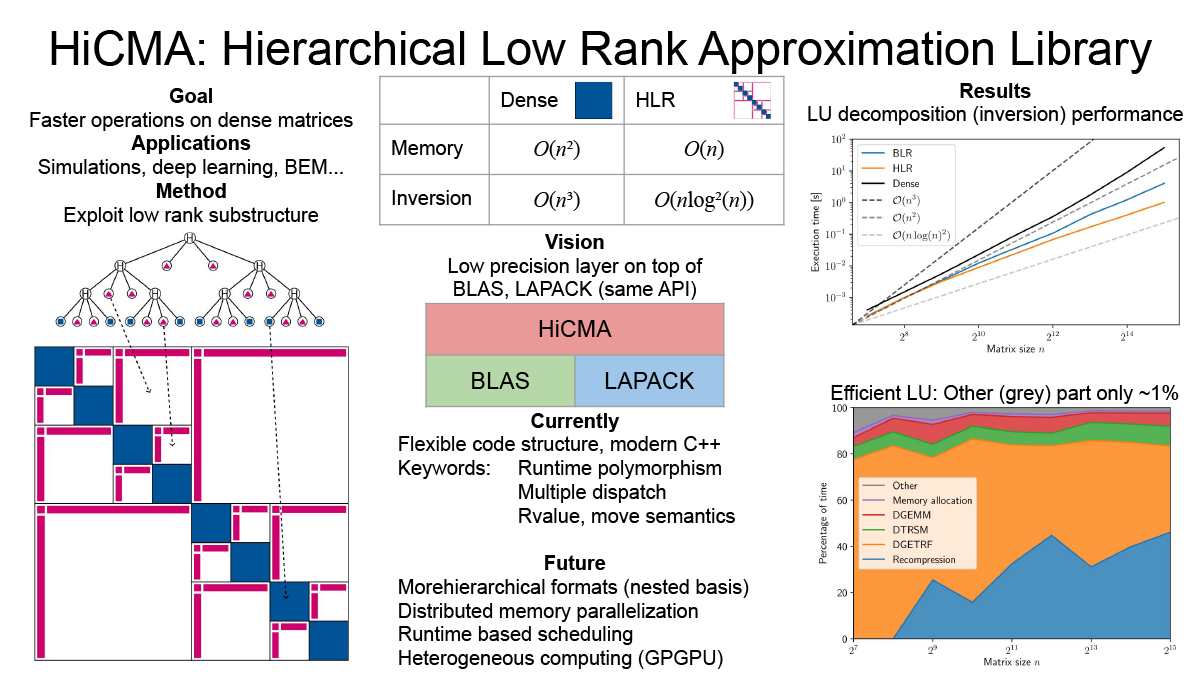## Research

### Thesis 2019

#### Efficient library for hierarchical low rank approximation (Peter Spalthoff)

Dense matrices have a quadratic memory complexity and many operations on them have cubic scaling. This makes them prohibitively expensive for large scale operations. In many applications (covariance matrices, BEM...) a substructure of low rank blocks is found. This substructure can be exploited to create an efficient compression of the matrix, called hierarchical low rank approximation. On the resulting so-called Hierarchical Matrices, which only have linear storage complexity, all arithmetic operations (multiplication, inversion...) can be defined. These operations are also much faster with cloes to linear complexity. We are working on a modern, flexible library with distributed memory parallelization on heterogeneous nodes.▲ Click image to enlarge

### Thesis 2018

#### Verification of speeding up using low precision arithmetic in convolutional neural network (Hiroki Naganuma)

The recent trend in convolutional neural networks (CNN) is to have deeper multilayered structures. While this improves the accuracy of the model, the amount of computation and the amount of data involved in learning and inference increases. In order to solve this problem, several techniques have been proposed to reduce the amount of data and the amount of computation by lowering the numerical precision of computation and data by utilizing the CNN's resistance to noise.

However, there is a lack of discussion on the relationship between parameter compression and speedup within each layer of the CNN.

In this research, we propose a method to speed up the inference by using half precision floating point SIMD instructions, by applying low precision to the learned model, in addition to reducing the data of the CNN model, and speeding up data access for layers that are computation-bound.

We examined the influence of CNN recognition accuracy, the speedup for each layer, and its reason, when we apply our method.▲ Click image to enlarge

#### Smoothing of the Objective Function in Stochastic Optimization for Large Scale Parallel Deep Learning (Hiroki Naganuma)

Classical learning theory states that when the number of parameters of the model is too large compared to the data, the model will overfit and the generalization performance deteriorates. However, it has been empirically shown that deep neural networks (DNN) can achieve high generalization capability by training with extremely large amount of data and model parameters, which exceeds the predictions of classical learning theory. One drawback of this is that training of DNN requires enormous calculation time. Therefore, it is necessary to reduce the training time through large scale parallelization. Straightforward data-parallelization of DNN degrades convergence and generalization. In the present work, we investigate the possibility of using second order methods to solve this generalization gap in large-batch training. This is motivated by our observation that each mini-batch becomes more statistically stable, and thus the effect of considering the curvature plays a more important role in large-batch training. We have also found that naively adapting the natural gradient method causes the generalization performance to deteriorate further due to the lack of regularization capability. We propose an improved second order method by smoothing the loss function, which allows second order methods to generalize as well as mini-batch SGD.▲ Click image to enlarge